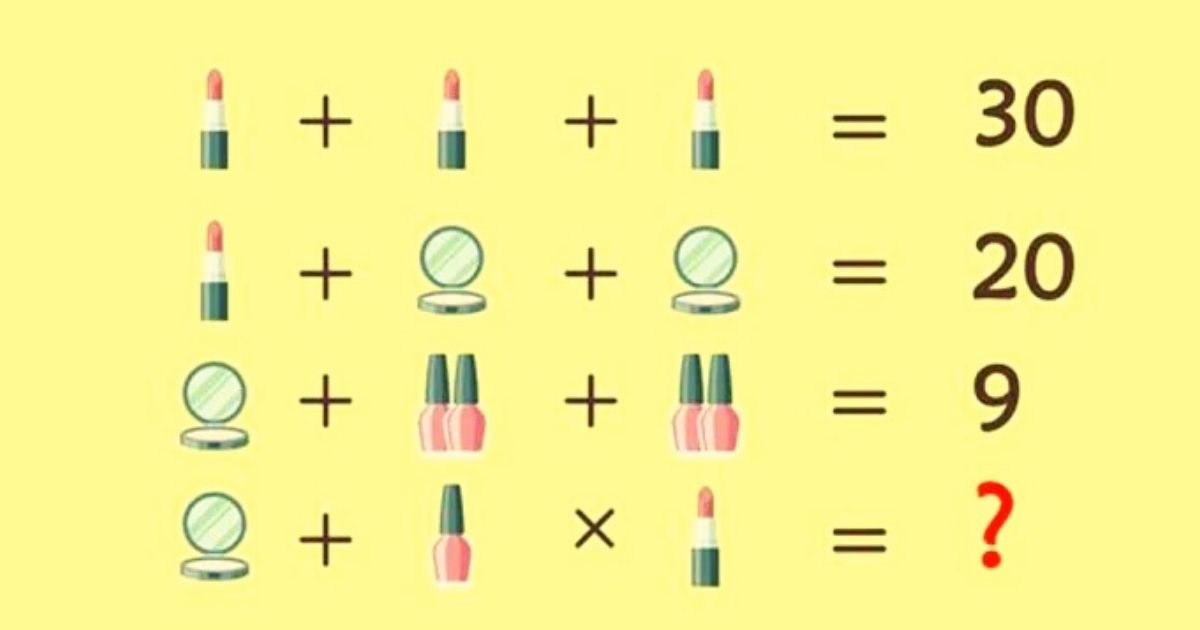# Can You Solve This Confusing Math Puzzle That’s Been Baffling The Internet

After people were challenged to find the proper answer to a perplexing arithmetic conundrum, the internet went wild.Though the math issue in question appears to be straightforward at first glance, bear in mind that it contains a number of traps that you must avoid. You must also follow the correct order of operations if you want to achieve the correct solution.

Let’s roll up our sleeves and get to work!

Put your brain cells to work and see if you can figure it out on your own before we disclose the correct answer and explain how to get there!

You’re probably confident that you’ve got the proper solution by now, so let’s look at the problem piece by piece!

To begin, assume that L represents lipstick, M represents mirror, and N represents nail polish.

FIRST LINE: L + L + L = 30, therefore 3L = 30 and L equals 10. The value of lipstick is 10.

SECOND LINE: L + M + M = 20, therefore 10 + 2M = 20 and M equals 5. The value of mirror is 5.

THIRD LINE:  M + 2N + 2N = 9, therefore 4N = 4 and N equals 1. The value of nail polish is 1.

FOURTH LINE: M + N x L = 5 + 1 x 10 = 15.Keep in mind that you must execute multiplication before addition to arrive at the right result since the former takes precedence. Also, while determining the worth of nail polish, be cautious!